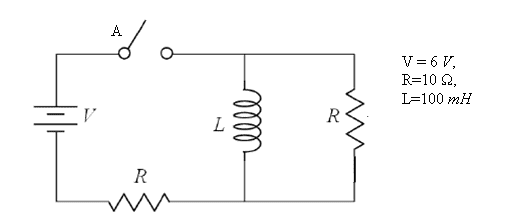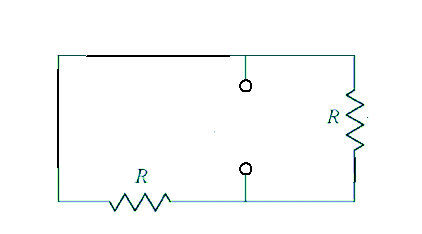# Time constant for the current through the inductor

## Homework Statementhttp://i.imgur.com/wjD8Y.png

In the circuit below, V = 6 volts, R = 10 ohms, L = 100 mH. The switch has been open for a long time. Then, at time t = 0, the switch is closed.

What is the time constant for the current through the inductor?

2L / R

TAU=l/R

## The Attempt at a Solution

I am not sure how do I find the equvialent resistance in this case.

Last edited:

Kill the source (short the voltage supply) and remove the inductor. Find the equivalent resistance at the terminals where the inductor was.Equvialent resistance is 2r then time constant should be L/2R?

Thanks

gneill
Mentor
Equvialent resistance is 2r then time constant should be L/2R?